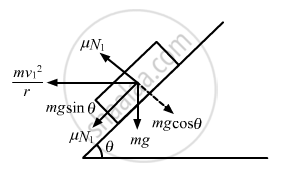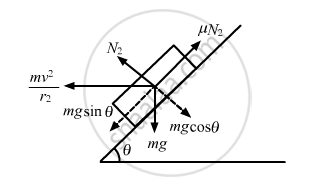Share

# A Turn of Radius 20 M is Banked for the Vehicles Going at a Speed of 36 Km/H. If the Coefficient of Static Friction Between the Road and the Tyre is 0.4, - Physics

ConceptCircular Motion

#### Question

A turn of radius 20 m is banked for the vehicles going at a speed of 36 km/h. If the coefficient of static friction between the road and the tyre is 0.4, what are the possible speeds of a vehicle so that it neither slips down nor skids up?

#### Solution

Given:
Speed of vehicles = v = 36 km/hr = 10 m/s
Radius = r = 20 m
Coefficient of static friction = μ = 0.4
Let the road be banked with an angle $\theta$

We have :

$\theta = \tan^{- 1} \frac{\text{v}^2}{\text{rg}}$

$= \tan^{- 1} \frac{100}{20 \times 10}$

$= \tan^{- 1} \left( \frac{1}{2} \right)$

$\Rightarrow \text{tan } \theta = 0 . 5$When the car travels at the maximum speed, it slips upward and μN1 acts downward.
Therefore we have :

$\text{ N}_1 - \text{mg}\cos\theta - \frac{\text{mv}_1^2}{r}\sin\theta = 0 . . . \left( \text{i} \right)$

$\mu \text{N}_1 + \text{mg }\sin\theta - \frac{\text{mv}_1^2}{r}\cos\theta = 0 . . . \left(\text{ ii} \right)$

On solving the above equations, we get :

$\text{v}_1 = \sqrt{\text{rg}\frac{\mu + \tan\theta}{1 - \mu\tan\theta}}$

$= \sqrt{20 \times 10 \times \frac{0 . 9}{0 . 8}}$

$= 15 \text{ m/s = 54 km/hr }$Similarly, for the other case, it can be proved that :

$\text{v}_2 = \sqrt{\text{rg}\frac{\text{tan}\theta - \mu}{\sqrt{1 - \mu \text{ tan }\theta}}}$

$= \sqrt{20 \times 10 \times \frac{0 . 1}{1 . 2}}$

$= 4 . 08 \text{ m/s = 14 . 7 km/hr}$

Thus, the possible speeds are between 14.7 km/hr and 54 km/hr so that the car neither slips down nor skids up.

Is there an error in this question or solution?

#### APPEARS IN

Solution A Turn of Radius 20 M is Banked for the Vehicles Going at a Speed of 36 Km/H. If the Coefficient of Static Friction Between the Road and the Tyre is 0.4, Concept: Circular Motion.
S